#DAY 29
0
AI & Data

## 自訂策略玩台車(Cartpole)

1. 台車距離中心點大於 2.4 單位就算輸了，所以設定每次行動採一左一右，盡量不離中心點https://ithelp.ithome.com.tw/markdown。
2. 由於台車平衡桿角度偏差12度以上也算輸，所以設定平衡桿角度偏右8度以上，就往右前進，直到角度偏右小於8度。
3. 反之，偏左也是類似處理。

1. 首先建立 Agent 類別，擬定策略，act 函數實現以上邏輯。
``````class Agent:
# 初始化
def __init__(self):
self.direction = left
self.last_direction=right

# 自訂策略
def act(self, observation):
# cart_position：台車位置(Cart Position)
# cart_velocity：台車速度(Cart Velocity)
# pole_angle：平衡桿角度(Pole Angle)
# pole_velocity：平衡桿速度(Pole Velocity At Tip)
cart_position, cart_velocity, pole_angle, pole_velocity = observation

'''
行動策略：
1. 設定每次行動採一左一右，盡量不離中心點。
2. 平衡桿角度偏右8度以上，就往右前進，直到角度偏右小於8度。
3. 反之，偏左也是同樣處理。
'''
if pole_angle < math.radians(max_angle) and pole_angle > math.radians(-max_angle):
self.direction = (self.last_direction + 1) % 2
elif pole_angle >= math.radians(max_angle):
self.direction = right
else:
self.direction = left

self.last_direction = self.direction

return self.direction
``````
1. 引用上述類別，將隨機策略修改為上述行動策略。
``````agent = Agent()
while True:
# 依策略行動
action = agent.act(observation) #env.action_space.sample()
``````
1. 實驗結果如下：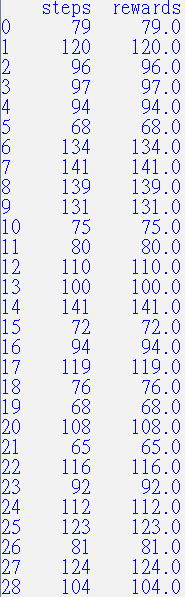• 它不會自我學習，玩再多次，也不會逐漸進步。
• 它沒有通用性(Generalization)，此策略邏輯沒辦法應用在其他遊戲，更不用說其他領域。

## 強化學習的理論基礎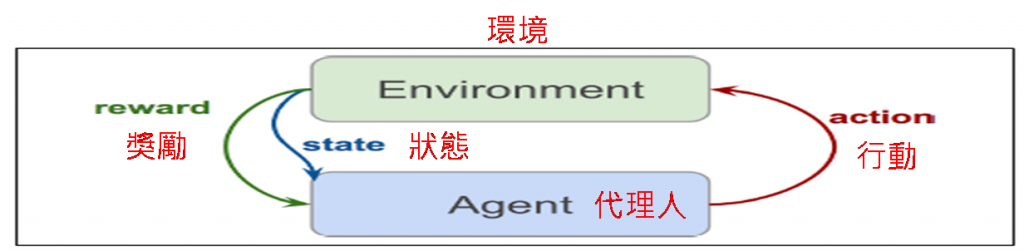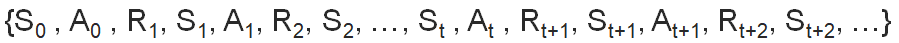### 報酬(Return)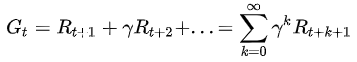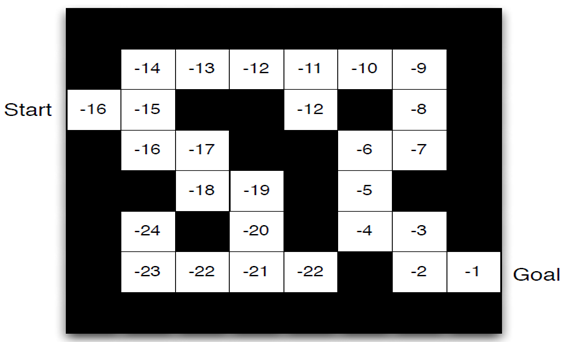### 狀態值函數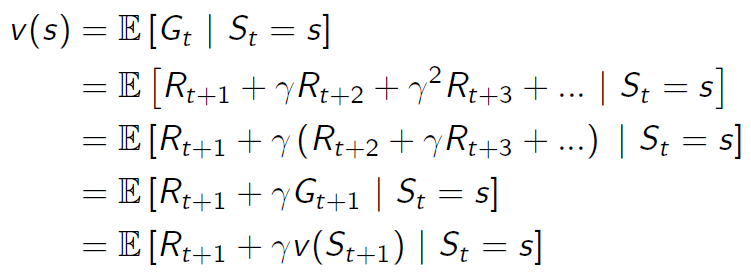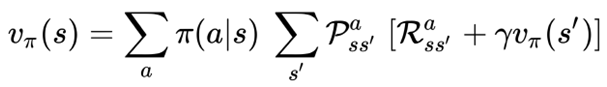• P：狀態轉移機率矩陣，是各種狀態之間轉換的機率，譬如打棒球，擊中後可能40%機率往前飛，30%往右偏，30%往左偏。
• π(a|s)：策略機率矩陣，是在狀態s時，採取各種行動(a)的機率，譬如打棒球，在已經兩好球後，打擊手可能 80% 會揮棒，20% 不揮棒。
• 乍看這個公式很奇怪，當前狀態值函數要由下一個狀態值函數算出來，在目前狀態下，我們怎麼知道下一個狀態值函數? 其實我們只要到達過終點一次，過程中的任何一狀態的值函數都可以由終點逆算回去，得到每一個狀態值函數，所以，當前狀態值函數由下一個狀態值函數推算出來就不奇怪了。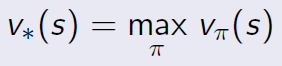### 行動值函數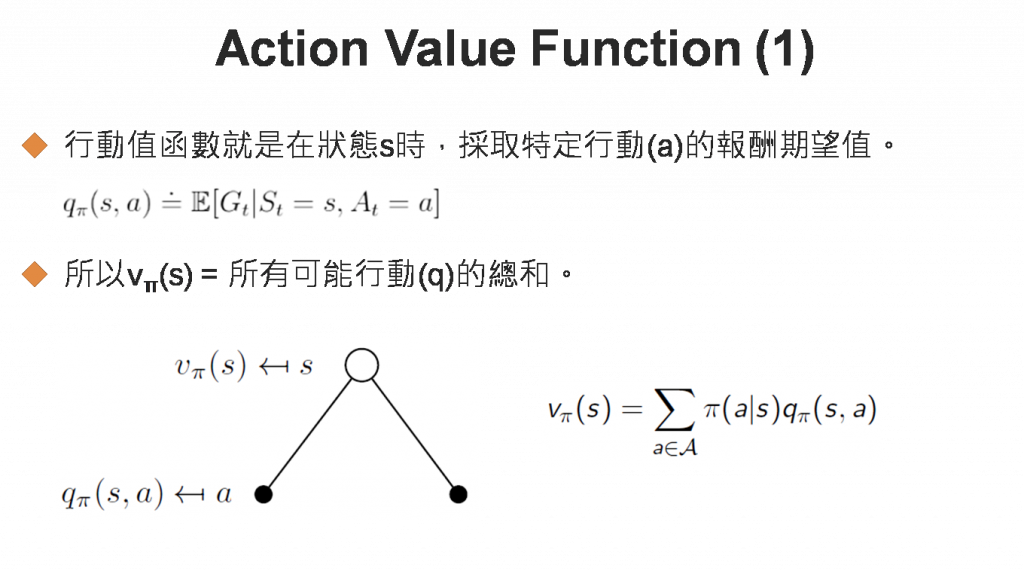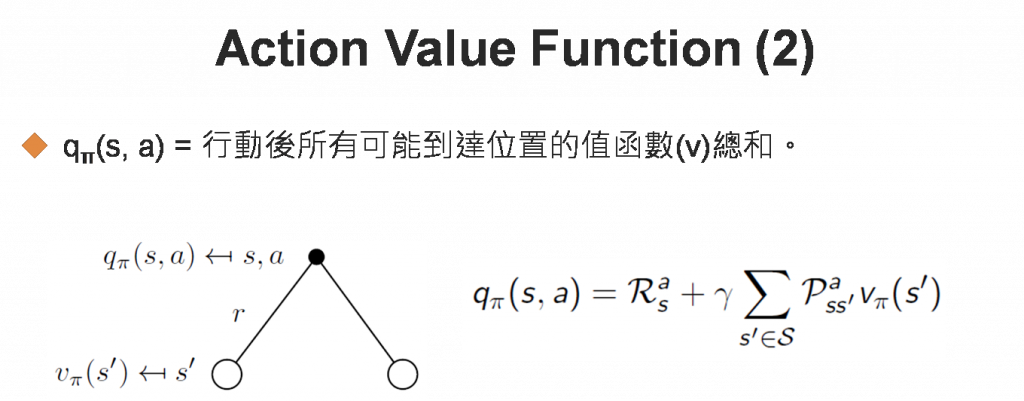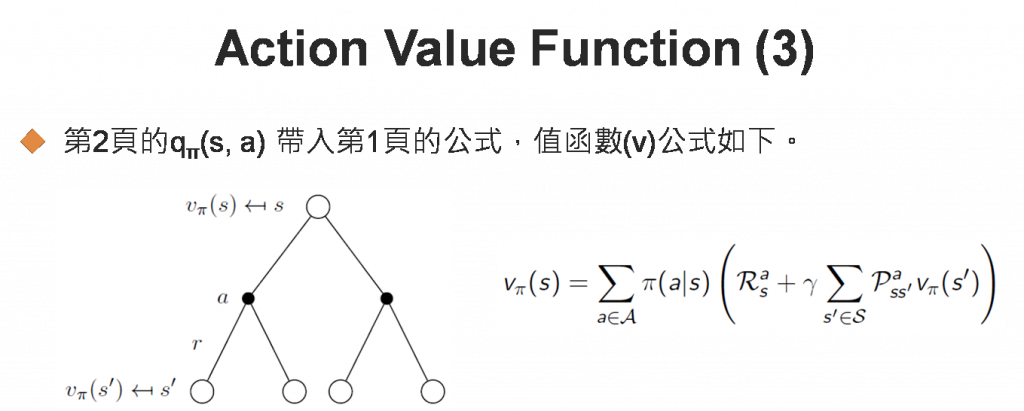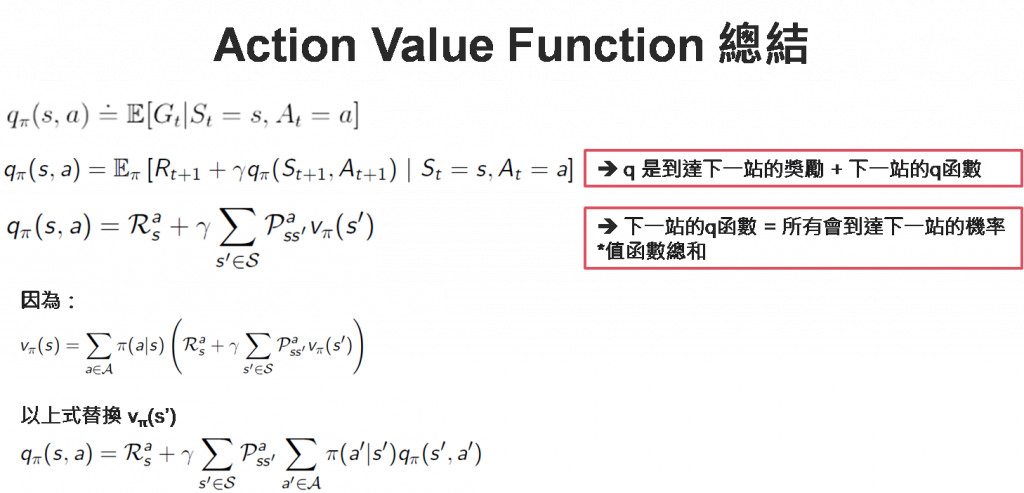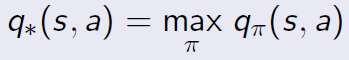## 策略迭代

(1) 策略評估(Policy evaluation)：先使用確定性的(deterministic)策略π(a|s)，估計每一個位置的狀態值函數，確定性的(deterministic)策略通常是一機率分配，如均勻(Uniform)分配、Poisson分配...等。
(2) 策略改善(Policy improvement)：再依照貪婪(Greedy)或其他戰術，選擇最有利的位置行動。在每個位置走下一步後，回到步驟(1)，重新計算所有位置的狀態值函數。一直迭代下去，直到策略不再變動為止，即最佳行動的選擇與目前策略完全一致。## 策略評估範例1. 左上方為起點，右下方為終點。
2. 每走一步，獎勵為-1，除非是起點或終點。
3. 若走一步會出迷宮，則保持在原位置。
4. 採隨機策略，往上、下、左、右走，機率均等(0.25)。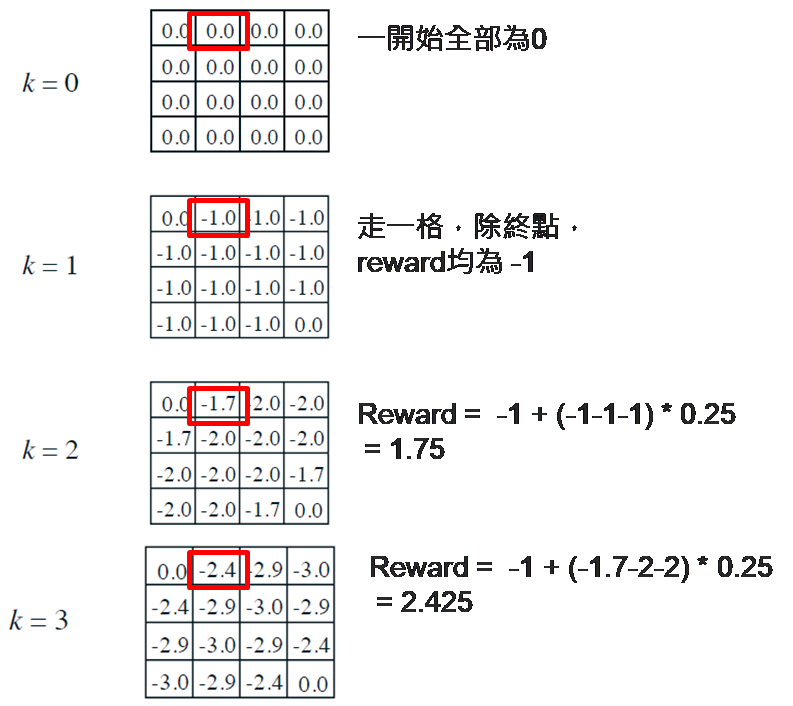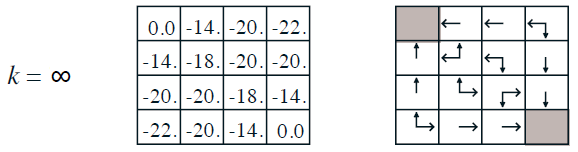## 策略評估實作

1. 載入套件。
``````from IPython.core.debugger import set_trace
import numpy as np
import pprint
import sys
from lib.envs.gridworld import GridworldEnv
``````
1. 載入遊戲。
``````pp = pprint.PrettyPrinter(indent=2)
env = GridworldEnv()
``````
1. 定義策略評估函數。
``````def policy_eval(policy, env, discount_factor=1.0, theta=0.00001):
"""
policy: [S, A] shaped matrix representing the policy.
env.P：狀態轉移機率矩陣
env.P[s][a]：(prob, next_state, reward, done) 陣列
env.nS：狀態總數
env.nA：行動總數
theta: 狀態值函數變動 <= theta，即停止進行。
discount_factor: 獎勵折扣率(Gamma)

傳回【狀態值函數】
"""
# 一開始【狀態值函數】均為 0
V = np.zeros(env.nS)
while True:
delta = 0
# For each state, perform a "full backup"
for s in range(env.nS):
v = 0
# 執行每一種可能的行動
for a, action_prob in enumerate(policy[s]):
# 行動可能到達的每一種狀態
for  prob, next_state, reward, done in env.P[s][a]:
# 計算【狀態值函數】期望值，參考圖三. 狀態值函數公式
v += action_prob * prob * (reward + discount_factor * V[next_state])
# 找出每一個位置的這一輪與上一輪狀態值函數差異最大者
delta = max(delta, np.abs(v - V[s]))
V[s] = v
# 最大差異 < 事先設定值 theta，則停止評估
if delta < theta:
break
return np.array(V)
``````
1. 測試。
``````# 隨機策略，每一個位置往上、下、左、右走的機率均為 0.25。
random_policy = np.ones([env.nS, env.nA]) / env.nA
# 策略評估
v = policy_eval(random_policy, env)
``````
1. 顯示最後結果，如下圖，與圖七【迷宮遊戲最終的狀態值函數】結果非常相近。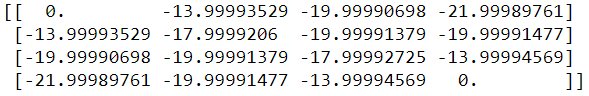## 策略迭代實作

1. 定義策略改善函數，先更新策略評估，再選擇最佳行動。
``````def policy_improvement(env, policy_eval_fn=policy_eval, discount_factor=1.0):
"""
policy_eval_fn: 策略評估函數。
discount_factor: 獎勵折扣率(Gamma)

Returns:
傳回 (policy, V) 陣列.
- policy：是最佳策略, [S, A] 二為矩陣，含每個狀態/行動組合的機率分配.
- V：狀態值函數。

"""

# 走下一步的處理，計算行動值函數
def one_step_lookahead(state, V):
"""
state: 狀態值
V: 狀態值函數

傳回【行動值函數】
"""
A = np.zeros(env.nA)
# 圖四. 行動值函數公式
for a in range(env.nA):
for prob, next_state, reward, done in env.P[state][a]:
A[a] += prob * (reward + discount_factor * V[next_state])
return A

# 隨機策略，每一個位置往上、下、左、右走的機率均為 0.25。
policy = np.ones([env.nS, env.nA]) / env.nA

while True:
# 策略評估
V = policy_eval_fn(policy, env, discount_factor)

# 策略是否趨於穩定的旗標
policy_stable = True

# 每一個狀態均採貪婪戰術
for s in range(env.nS):
# 貪婪戰術
chosen_a = np.argmax(policy[s])

# 走下一步的處理，計算行動值函數
action_values = one_step_lookahead(s, V)
# 找出最大行動值函數
best_a = np.argmax(action_values)

# 貪婪戰術 不等於 最大行動值函數，繼續迭代
if chosen_a != best_a:
policy_stable = False
policy[s] = np.eye(env.nA)[best_a]

# 策略趨於穩定就結束訓練
if policy_stable:
return policy, V
``````
1. 測試：顯示策略，即在每個狀態/行動組合的機率分配。
``````policy, v = policy_improvement(env)
print("Policy Probability Distribution:")
print(policy)
``````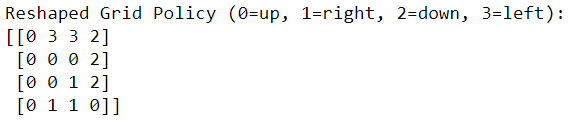## 值迭代

1. 定義值迭代函數，先更新狀態值函數。
``````def value_iteration(env, theta=0.0001, discount_factor=1.0):
"""
env.P：狀態轉移機率矩陣
env.P[s][a]：(prob, next_state, reward, done) 陣列
env.nS：狀態總數
env.nA：行動總數
theta: 狀態值函數變動 <= theta，即停止進行。
discount_factor: 獎勵折扣率(Gamma)

Returns:
傳回 (policy, V) 陣列.
- policy：是最佳策略, [S, A] 二為矩陣，含每個狀態/行動組合的機率分配.
- V：狀態值函數。
"""

def one_step_lookahead(state, V):
"""
state: 狀態值
V: 狀態值函數

傳回【行動值函數】
"""
A = np.zeros(env.nA)
for a in range(env.nA):
for prob, next_state, reward, done in env.P[state][a]:
A[a] += prob * (reward + discount_factor * V[next_state])
return A

V = np.zeros(env.nS)
while True:
delta = 0
# 每一個狀態均採貪婪戰術
for s in range(env.nS):
# 走下一步的處理，計算行動值函數
A = one_step_lookahead(s, V)
# 找出最大行動值函數
best_action_value = np.max(A)
# 找出每一個位置的這一輪與上一輪狀態值函數差異最大者
delta = max(delta, np.abs(best_action_value - V[s]))
# 更新值函數
V[s] = best_action_value
# 最大差異 < 事先設定值 theta，則停止評估
if delta < theta:
break

# 再作一次，產生 policy
policy = np.zeros([env.nS, env.nA])
for s in range(env.nS):
# 走下一步的處理，計算行動值函數
A = one_step_lookahead(s, V)
best_action = np.argmax(A)
# 設定目前狀態下最佳的行動
policy[s, best_action] = 1.0

return policy, V
``````
1. 測試：顯示策略，即在每個狀態/行動組合的機率分配。
``````policy, v = value_iteration(env)

print("Policy Probability Distribution:")
print(policy)
``````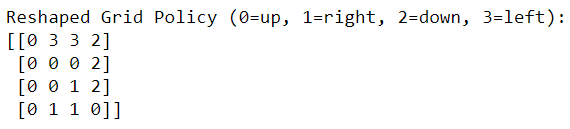## 結論

1. 狀態轉移機率矩陣是已知的：利用有限的歷史資料統計，可能是不足的，例如天氣預報，預測準確率不高。
2. 策略機率矩陣是已知的：同樣的問題。
3. 要走過每個位置才能算出狀態值函數，許多遊戲狀態很多，很難一一經歷到，例如圍棋，每個位置有3種狀態(空白/黑子/白子)，棋面總共有19x19個位置，狀態空間總共有 3 的 19x19 次方，約為10的172次方，花個幾百年也走不遍每一個位置。

• Dynamic Programming
• Monte Carlo Prediction
• Monte Carlo Control with Epsilon-Greedy Policies
• Monte Carlo Off-Policy Control with Importance Sampling
• SARSA (On Policy TD Learning)
• Q-Learning (Off Policy TD Learning)
• Q-Learning with Linear Function Approximation
• Deep Q-Learning for Atari Games
• Double Deep-Q Learning for Atari Games
• Deep Q-Learning with Prioritized Experience Replay (WIP)
• Policy Gradient: REINFORCE with Baseline
• Policy Gradient: Actor Critic with Baseline
• Policy Gradient: Actor Critic with Baseline for Continuous Action Spaces
• Deterministic Policy Gradients for Continuous Action Spaces (WIP)
• Deep Deterministic Policy Gradients (DDPG) (WIP)
• Asynchronous Advantage Actor Critic (A3C)